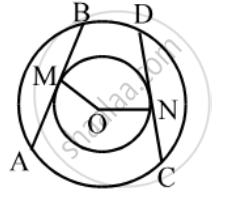# In two concentric circles, prove that all chords of the outer circle which touch the inner circle are of equal length. - Mathematics

Sum

In two concentric circles, prove that all chords of the outer circle which touch the inner circle are of equal length.

#### Solution

Let AB and CD be two chords of the circle which touch the inner circle at M and N respectively.Then, we have to prove that

AB = CD

Since AB and CD are tangents to the smaller circle.

∴ OM = ON = Radius of the smaller circle

Thus, AB and CD are two chords of the larger circle such that they are equidistant from the centre. Hence, AB = CD

Concept: Concept of Circle - Centre, Radius, Diameter, Arc, Sector, Chord, Segment, Semicircle, Circumference, Interior and Exterior, Concentric Circles
Is there an error in this question or solution?